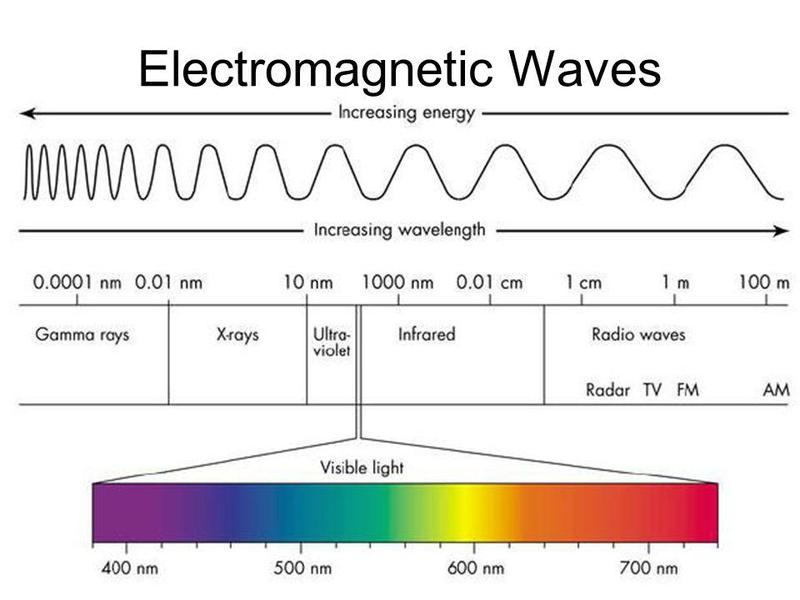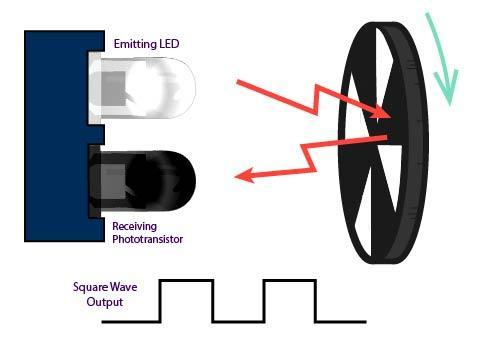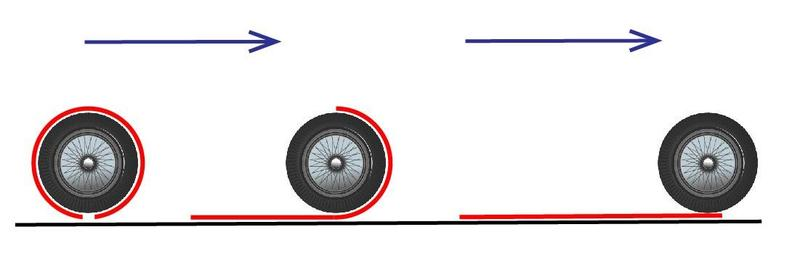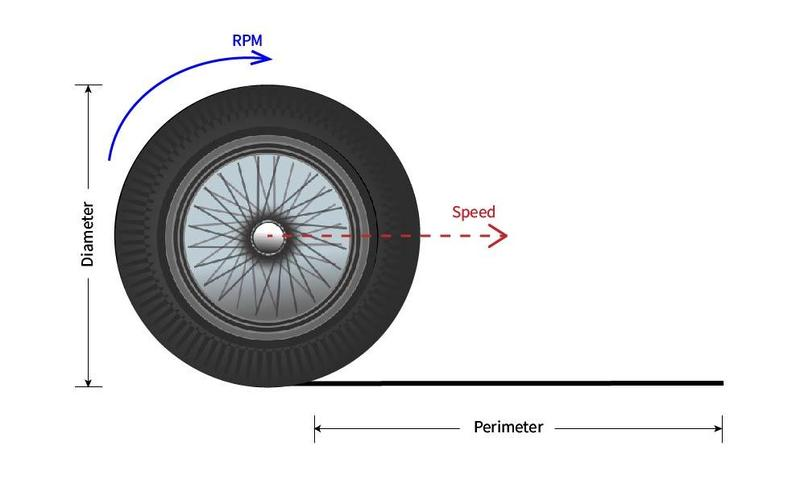Infrared

### What is Infrared?

Infrared is a type of electromagnetic wave, similar to x-rays and microwaves. Electromagnetic (EM) waves are also a form of light. EM waves bounces off objects and into our eyes, allowing us to see colors. Each color we see has a different wavelength where wavelength is the distance between two crests (the highest point) on a wave. All of the different EM waves have different wavelengths with shorter wavelengths having higher energy and longer wavelengths having less energy. This broad range of waves makes up the Electromagnetic Spectrum.Electromagnetic Spectrum

The EM waves humans can see are only a small fraction of all the EM waves in the universe; the wavelengths we can see are between 400 - 700 nanometers (about nine times smaller than a human hair) while the entire EM spectrum goes from Gamma rays with wavelengths of about 0.0001 nanometers to Radio waves with wavelengths of about 100 meters.

Infrared (IR) light has a longer wavelength than the light visible to humans. It ranges from about 700 nanometers to .1 millimeters. This means we can't see infrared, but it's there and you can feel it as heat! Because humans can't see IR, it is commonly used in remote controls. In a TV remote for example, when you click the button to turn the volume up the microprocessor in the remote converts that action into a binary code that is then emitted from an IR emitting LED to and IR receiver on the TV. This code is sent out in pulses of light that the TV's own microprocessor can then detect and decode, resulting in the volume increasing.

Infrared radiation is also a way of transferring heat and makes up almost half of the sun's energy. While some energy from the sun bounces off objects and allows us to see colors, most of the energy is absorbed by objects around us.

This occurrence allows for one of the most important uses for infrared light - bolometers. A device that measures IR energy named after the Greek word for a ray of light, bolē. The IR light emitted from objects can be detected by electronic sensors such as a temperature-sensitive resistor called a thermistor. An increase in heat or IR energy lowers the resistance of a thermistor. This change in resistance causes a change in voltage. Much like the sensors in past Thimble kits, we can measure this change and covert it to a temperature reading.

### Infrared Encoder

So how are we going to use infrared light? A common way to accurately measure how far a motor has turned is an infrared encoder. The line finder module in the WiFi Robot 1 kit has one IR emitting LED (the clear LED) and an IR sensitive phototransistor (the dark bulb). The clear bulb emits an IR beam that can bounce back and be detected by the dark bulb. The emitter constantly sends an IR signal. When that signal hits a reflective surface or light colored surface, it bounces back and into the phototransistor. High causes a measurable voltage change and HIGH signal output from the sensor. If the IR signal doesn't hit anything or hits a dark surface, the signal doesn't make it to the phototransistor and the sensor outputs a LOW signal. We use this property in the encoding wheel which has patches of black and white. When the sensor goes from LOW to HIGH to LOW, we know that the wheel is turning. How quickly the LOW to HIGH to LOW changes can be directly corelated to the speed of the wheel.As the encoding wheel turns the IR ray bounces back and results in a square wave input

### Using IR for Measurements

Once we are able to count the number of turns in the motor using the IR sensor and encoder wheels, we can also calculate the velocity using a little bit of geometry.

The circumference of a circle is pi times diameter. When the wheel fully turns once, it goes a distance thats equal to its circumference.Wheel circumference

This means that the distance the robot travels is equal to the number of times the wheel completes one full rotation times the circumference.

To figure out how many ticks will get us a specific distance we can divide the 360 degrees in a circle by the circumference to see how far the wheel will travel per tick.

Multiplying this by the distance we want to go will tell us how many ticks to count to go that distance.

#### Velocity

In order to calculate velocity we need to know the RPM of the motor. RPM stands for "Rotations Per Minute" and is a number that indicates how many times the motor turns one full rotation in one minute. By knowing how many times the motor rotates per minute we can use the distance it travels in one rotation (its circumference) to find its velocity with the formula velocity = circumference * (rpm / 60) .

We divide by 60 to get rotations per seconds (rps) which gives us the velocity in a standard unit such as meters per second (m/s).Wheel speed illustration

Now that you know what IR is and how we can use it for many calculations... Let's get on to building your robot!